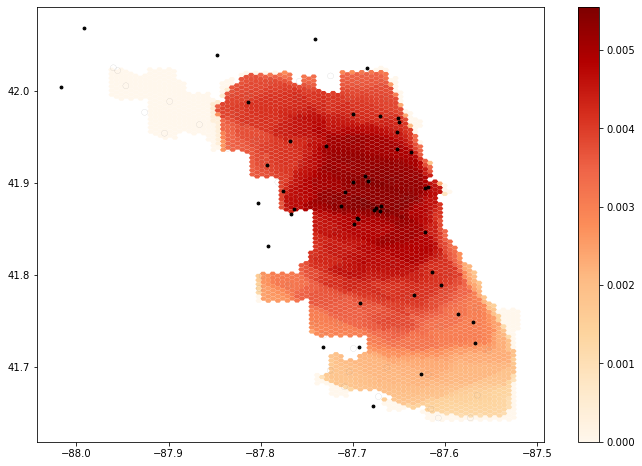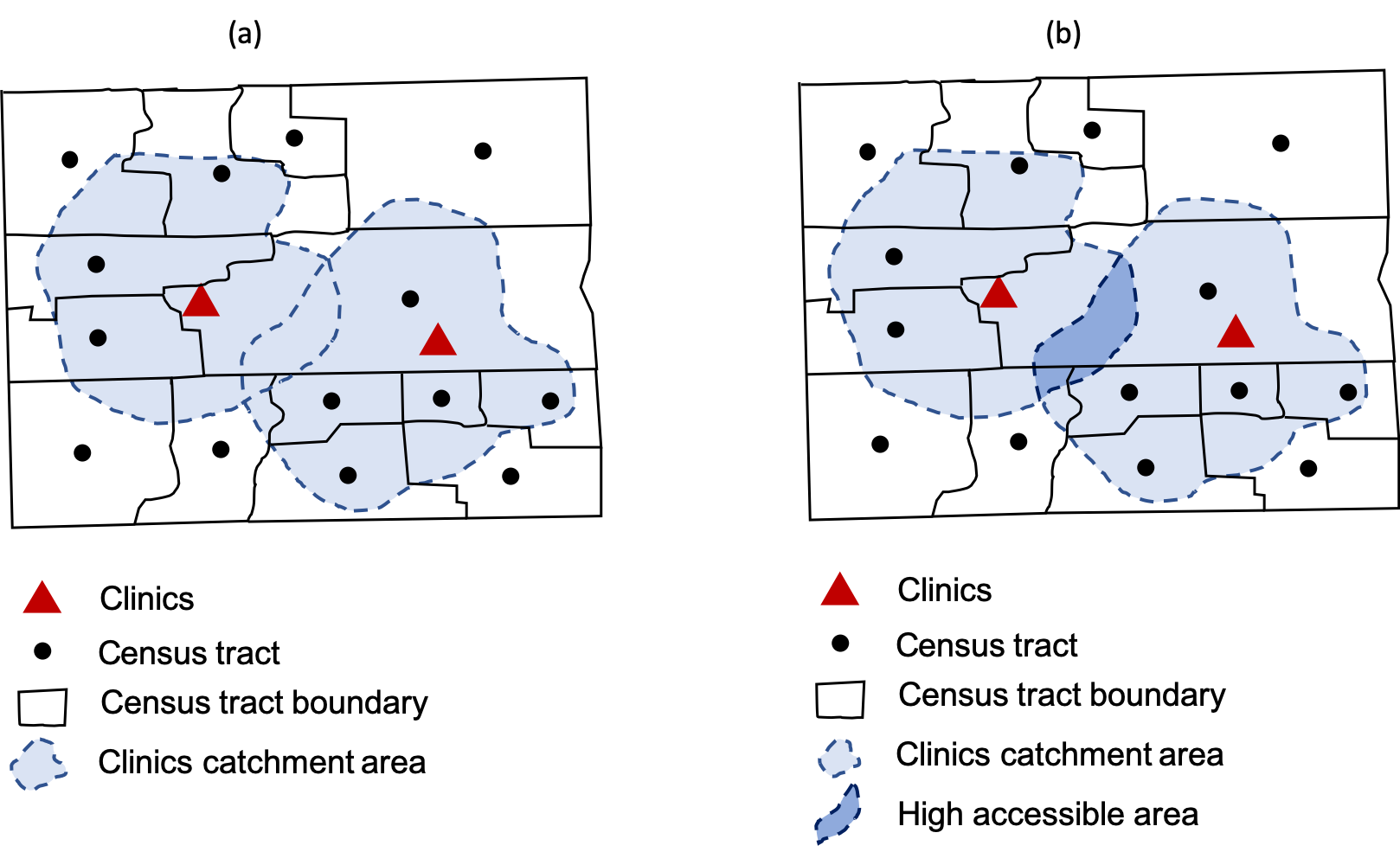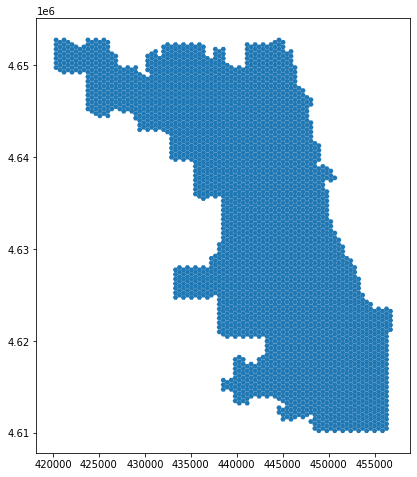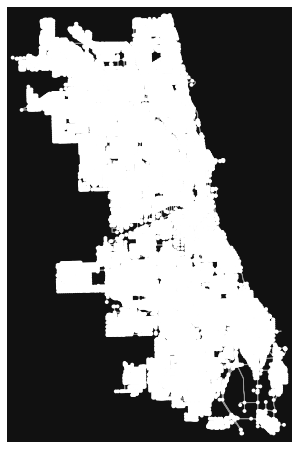Rapidly Measuring Spatial Accessibility of COVID-19 Healthcare Resources: A Case Study of Illinois, USA

**Author**: Jeon-Young Kang 1,2, Alexander Michels 1,3, Fanzheng Lyu1,2, Shaohua Wang1,2 , Nelson Agbodo4, Vincent Freeman5, Shaowen Wang1,2,3,*

1 CyberGIS Center for Advanced Digital and Spatial Studies, University of Illinois at UrbanaChampaign, Urbana, Illinois
2 Department of Geography and Geographic Information Science, University of Illinois at Urbana-Champaign, Urbana, Illinois
3 Illinois Informatics Institute, University of Illinois at Urbana-Champaign, Urbana, Illinois
4 Division of Health Data and Policy, Illinois Department of Public Health, Springfield, Illinois
5 Division of Epidemiology and Biostatistics, School of Public Health, University of Illinois at Chicago, Chicago, Illinois

* Corresponding author: shaowen@illinois.edu

### You can checkout the paper here: https://doi.org/10.1186/s12942-020-00229-x¶### Reference¶

Kang, J. Y., Michels, A. C., Lyu, F., Wang, S., Agbodo, N., Freeman, V. L., & Wang, S. (2020). Rapidly Measuring Spatial Accessibility of COVID-19 Healthcare Resources: A Case Study of Illinois, USA. medRxiv https://doi.org/10.1101/2020.05.06.20093534

### Objectives¶

This aims to measure spatial access for people to hospitals in Illinois. The spatial accessibiilty is measured by the use of an enhanced two-step floating catchment area (E2FCA) method (Luo & Qi, 2009), which is an outcome of interactions between demands (i.e, # of potential patients; people) and supply (i.e., # of beds or physicians). As a result, the map of spatial accessibility will be produced. It identifies which regions need more healthcare resources, such as the number of beds or physicians. This notebook would serve as a guideline of which regions need more beds for fighting against COVID-19.

### Data¶

To perform the ESFCA method, three types of data are required, as follows: (1) road network, (2) population, and (3) hospital information. The road network can be obtained from the OpenStreetMap Python Library, called OSMNX. The population data is available on the American Community Survey. Lastly, hosptial information is also publically available on the Homelanad Infrastructure Foundation-Level Data.

### Method - An enhanced two-step floating catchment area (E2FCA)¶

The catchement area for each hospital will be delineated by travel times. People living in the overlappying regions by multiple hospitals' catchment area are more accessible to hospitals than people living in other places.### Codes¶

Import necessary libraries to run this model. See requirements.txt for the library versions used for this analysis.

In :
import pandas as pd
import numpy as np
import geopandas as gpd
import networkx as nx
import osmnx as ox
from shapely.geometry import Point, LineString, Polygon
import matplotlib.pyplot as plt
from tqdm import tqdm
import multiprocessing as mp
import folium, itertools, os, time, warnings
from IPython.display import display, clear_output

warnings.filterwarnings("ignore")


### Population and COVID-19 Cases Data by County¶

In :
pop_data = gpd.read_file('./data/PopData/Chicago_Tract.shp')

Out:
GEOID STATEFP COUNTYFP TRACTCE NAMELSAD Pop Unnamed_ 0 NAME OverFifty TotalPop geometry
0 17031010400 17 031 010400 Census Tract 104 5153 1538 Census Tract 104, Cook County, Illinois 1103 5153 POLYGON ((-87.66125 42.01288, -87.66125 42.012...
1 17031010600 17 031 010600 Census Tract 106 6271 438 Census Tract 106, Cook County, Illinois 1469 6271 POLYGON ((-87.67059 42.00537, -87.67046 42.005...
2 17031030200 17 031 030200 Census Tract 302 5444 2075 Census Tract 302, Cook County, Illinois 2018 5444 POLYGON ((-87.67062 41.99808, -87.67045 41.998...
3 17031030300 17 031 030300 Census Tract 303 3464 516 Census Tract 303, Cook County, Illinois 1097 3464 POLYGON ((-87.67501 41.99799, -87.67473 41.998...
4 17031030400 17 031 030400 Census Tract 304 2582 1520 Census Tract 304, Cook County, Illinois 860 2582 POLYGON ((-87.67471 41.99076, -87.67440 41.990...

### Hospital Data¶

Note that 999 is treated as a "NULL"/"NA" so these hospitals are filtered out. This data contains the number of ICU beds and ventilators at each hospital.

In :
hospitals = gpd.read_file('./data/HospitalData/Chicago_Hospital_Info.shp')

Out:
FID Hospital City ZIP_Code X Y Total_Bed Adult ICU Total Vent geometry
0 2 Methodist Hospital of Chicago Chicago 60640 -87.671079 41.972800 145 36 12 MULTIPOINT (-87.67108 41.97280)
1 4 Advocate Christ Medical Center Oak Lawn 60453 -87.732483 41.720281 785 196 64 MULTIPOINT (-87.73248 41.72028)
2 13 Evanston Hospital Evanston 60201 -87.683288 42.065393 354 89 29 MULTIPOINT (-87.68329 42.06539)
3 24 AMITA Health Adventist Medical Center Hinsdale Hinsdale 60521 -87.920116 41.805613 261 65 21 MULTIPOINT (-87.92012 41.80561)
4 25 Holy Cross Hospital Chicago 60629 -87.690841 41.770001 264 66 21 MULTIPOINT (-87.69084 41.77000)

### Generate and Plot Map of Hospitals¶

In :
m = folium.Map(location=[41.85, -87.65], tiles='cartodbpositron', zoom_start=10)
for i in range(0, len(hospitals)):
folium.CircleMarker(
location=[hospitals.iloc[i]['Y'], hospitals.iloc[i]['X']],
popup="{}{}\n{}{}\n{}{}".format('Hospital Name: ',hospitals.iloc[i]['Hospital'],
'Ventilators: ', hospitals.iloc[i]['Total Vent']),
color='grey',
fill=True,
fill_opacity=0.6,
legend_name = 'Hospitals'
legend_html =   '''<div style="position: fixed; width: 20%; heigh: auto;
bottom: 10px; left: 10px;
solid grey; z-index:9999; font-size:14px;
">&nbsp; Legend<br>'''

m

Out:
Make this Notebook Trusted to load map: File -> Trust Notebook

### Load and Plot Hexagon Grids (500-meter resolution)¶

In :
grid_file = gpd.read_file('./data/GridFile/Chicago_Grid.shp')
grid_file.plot(figsize=(8,8))

Out:
<AxesSubplot:>### Load and Plot the Street Network¶

In :
graphml_path = "data/Chicago_Network.graphml"
if not os.path.exists(graphml_path):
G = ox.graph_from_place('Chicago', network_type='drive') # pulling the drive network the first time will take a while
ox.save_graphml(G, graphml_path)
else:
ox.plot_graph(G)Out:
(<Figure size 576x576 with 1 Axes>, <AxesSubplot:>)

## "Helper" Functions¶

The functions below are needed for our analysis later, let's take a look!

### network_setting¶

Cleans the OSMNX network to work better with drive-time analysis.

First, we remove all nodes with 0 outdegree because any hospital assigned to such a node would be unreachable from everywhere. Next, we remove small (under 10 node) strongly connected components to reduce erroneously small ego-centric networks. Lastly, we ensure that the max speed is set and in the correct units before calculating time.

Args:

• network: OSMNX network for the spatial extent of interest

Returns:

• OSMNX network: cleaned OSMNX network for the spatial extent
In :
def network_setting(network):
_nodes_removed = len([n for (n, deg) in network.out_degree() if deg ==0])
network.remove_nodes_from([n for (n, deg) in network.out_degree() if deg ==0])
for component in list(nx.strongly_connected_components(network)):
if len(component)<10:
for node in component:
_nodes_removed+=1
network.remove_node(node)
for u, v, k, data in tqdm(G.edges(data=True, keys=True),position=0):
if 'maxspeed' in data.keys():
speed_type = type(data['maxspeed'])
if (speed_type==str):
if len(data['maxspeed'].split(','))==2:
data['maxspeed']=float(data['maxspeed'].split(','))
elif data['maxspeed']=='signals':
data['maxspeed']=35.0 # drive speed setting as 35 miles
else:
data['maxspeed']=float(data['maxspeed'].split())
else:
data['maxspeed']=float(data['maxspeed'].split())
else:
data['maxspeed']= 35.0 #miles
data['maxspeed_meters'] = data['maxspeed']*26.8223 # convert mile to meter
data['time'] = float(data['length'])/ data['maxspeed_meters']
print("Removed {} nodes ({:2.4f}%) from the OSMNX network".format(_nodes_removed, _nodes_removed/float(network.number_of_nodes())))
print("Number of nodes: {}".format(network.number_of_nodes()))
print("Number of edges: {}".format(network.number_of_edges()))
return(network)


### hospital_setting¶

Finds the nearest OSMNX node for each hospital.

Args:

• hospital: GeoDataFrame of hospitals
• G: OSMNX network

Returns:

• GeoDataFrame of hospitals with info on nearest OSMNX node
In :
def hospital_setting(hospitals, G):
hospitals['nearest_osm']=None
for i in tqdm(hospitals.index, desc="Find the nearest osm from hospitals", position=0):
hospitals['nearest_osm'][i] = ox.get_nearest_node(G, [hospitals['Y'][i], hospitals['X'][i]], method='euclidean') # find the nearest node from hospital location
print ('hospital setting is done')
return(hospitals)


### pop_centroid¶

Converts geodata to centroids

Args:

• pop_data: a GeodataFrame
• pop_type: a string, either "pop" for general population or "covid" for COVID-19 case data

Returns:

• GeoDataFrame of centroids with population data
In :
# to estimate the centroids of census tract / county
def pop_centroid (pop_data, pop_type):
pop_data = pop_data.to_crs({'init': 'epsg:4326'})
if pop_type =="pop":
pop_data=pop_data[pop_data['OverFifty']>=0]
if pop_type =="covid":
pop_data=pop_data[pop_data['cases']>=0]
pop_cent = pop_data.centroid # it make the polygon to the point without any other information
pop_centroid = gpd.GeoDataFrame()
i = 0
for point in tqdm(pop_cent, desc='Pop Centroid File Setting', position=0):
if pop_type== "pop":
pop = pop_data.iloc[i]['OverFifty']
code = pop_data.iloc[i]['GEOID']
if pop_type =="covid":
pop = pop_data.iloc[i]['cases']
code = pop_data.iloc[i].ZCTA5CE10
pop_centroid = pop_centroid.append({'code':code,'pop': pop,'geometry': point}, ignore_index=True)
i = i+1
return(pop_centroid)


### calculate_catchment_area¶

Calculates a catchment area of things within some distance of a point using a given metric.

Function first creates an ego-centric subgraph on the NetworkX road network starting with the nearest OSM node for the hospital and going out to a given distance as measured by the distance unit. We then calculate the convex hull around the nodes in the ego-centric subgraph and make it a GeoPandas object.

Args:

• G: OSMNX network
• nearest_osm: OSMNX road network node that is closest to the place of interest (hospital)
• distance: the max distance to include in the catchment area
• distance_unit: how we measure distance (used by ego_graph), we always use time

Returns:

• GeoDataFrame the catchment area.
In :
def calculate_catchment_area(G, nearest_osm, distance, distance_unit = "time"):
road_network = nx.ego_graph(G, nearest_osm, distance, distance=distance_unit)
nodes = [Point((data['x'], data['y'])) for node, data in road_network.nodes(data=True)]
polygon = gpd.GeoSeries(nodes).unary_union.convex_hull ## to create convex hull
polygon = gpd.GeoDataFrame(gpd.GeoSeries(polygon)) ## change polygon to geopandas
polygon = polygon.rename(columns={0:'geometry'}).set_geometry('geometry')
return polygon.copy(deep=True)


### hospital_measure_acc¶

Measures the effect of a single hospital on the surrounding area. (Uses calculate_catchment_area)

Args:

• _thread_id: int used to keep track of which thread this is
• hospital: Geopandas dataframe with information on a hospital
• pop_data: Geopandas dataframe with population data
• distances: Distances in time to calculate accessibility for
• weights: how to weight the different travel distances

Returns:

• Tuple containing:
• GeoDataFrame of catchment areas with key stats
In :
def hospital_measure_acc (_thread_id, hospital, pop_data, distances, weights):
##distance weight = 1, 0.68, 0.22
polygons = []
for distance in distances:
polygons.append(calculate_catchment_area(G, hospital['nearest_osm'],distance))
for i in reversed(range(1, len(distances))):
polygons[i] = gpd.overlay(polygons[i], polygons[i-1], how="difference")

num_pops = []
for j in pop_data.index:
point = pop_data['geometry'][j]
for k in range(len(polygons)):
if len(polygons[k]) > 0: # to exclude the weirdo (convex hull is not polygon)
if (point.within(polygons[k].iloc["geometry"])):
num_pops.append(pop_data['pop'][j]*weights[k])
total_pop = sum(num_pops)
for i in range(len(distances)):
polygons[i]['time']=distances[i]
polygons[i]['total_pop']=total_pop
polygons[i]['hospital_icu_beds'] = float(hospital['Adult ICU'])/polygons[i]['total_pop'] # proportion of # of beds over pops in 10 mins
polygons[i]['hospital_vents'] = float(hospital['Total Vent'])/polygons[i]['total_pop'] # proportion of # of beds over pops in 10 mins
polygons[i].crs = { 'init' : 'epsg:4326'}
polygons[i] = polygons[i].to_crs({'init':'epsg:32616'})
print('\rCatchment for hospital {:4.0f} complete'.format(_thread_id), end="")
return(_thread_id, [ polygon.copy(deep=True) for polygon in polygons ])


### measure_acc_par¶

Parallel implementation of accessibility measurement.

Args:

• hospitals: Geodataframe of hospitals
• pop_data: Geodataframe containing population data
• network: OSMNX street network
• distances: list of distances to calculate catchments for
• weights: list of floats to apply to different catchments
• num_proc: number of processors to use.

Returns:

• Geodataframe of catchments with accessibility statistics calculated
In :
def hospital_acc_unpacker(args):
return hospital_measure_acc(*args)

def measure_acc_par (hospitals, pop_data, network, distances, weights, num_proc = 4):
catchments = []
for distance in distances:
catchments.append(gpd.GeoDataFrame())
pool = mp.Pool(processes = num_proc)
hospital_list = [ hospitals.iloc[i] for i in range(len(hospitals)) ]
results = pool.map(hospital_acc_unpacker, zip(range(len(hospital_list)), hospital_list, itertools.repeat(pop_data), itertools.repeat(distances), itertools.repeat(weights)))
pool.close()
results.sort()
results = [ r for r in results ]
for i in range(len(results)):
for j in range(len(distances)):
catchments[j] = catchments[j].append(results[i][j], sort=False)
return catchments


### overlap_calc¶

Calculates and aggregates accessibility statistics for one catchment on our grid file.

Args:

• poly: GeoDataFrame representing a catchment area
• grid_file: a GeoDataFrame representing our grids
• weight: the weight to applied for a given catchment
• service_type: the service we are calculating for: ICU beds or ventilators

Returns:

• Tuple containing:
• Counter object (dictionary for numbers) with aggregated stats by grid ID number
In :
from collections import Counter
def overlap_calc(_id, poly, grid_file, weight, service_type):
value_dict = Counter()
if type(poly.iloc[service_type])!=type(None):
value = float(poly[service_type])*weight
intersect = gpd.overlay(grid_file, poly, how='intersection')
intersect['overlapped']= intersect.area
intersect['percent'] = intersect['overlapped']/intersect['area']
intersect=intersect[intersect['percent']>=0.5]
intersect_region = intersect['id']
for intersect_id in intersect_region:
try:
value_dict[intersect_id] +=value
except:
value_dict[intersect_id] = value
return(_id, value_dict)

def overlap_calc_unpacker(args):
return overlap_calc(*args)


### overlapping_function¶

Calculates how all catchment areas overlap with and affect the accessibility of each grid in our grid file.

Args:

• grid_file: GeoDataFrame of our grid
• catchments: GeoDataFrame of our catchments
• service_type: the kind of care being provided (ICU beds vs. ventilators)
• weights: the weight to apply to each service type
• num_proc: the number of processors

Returns:

• Geodataframe - grid_file with calculated stats
In :
def overlapping_function (grid_file, catchments, service_type, weights, num_proc = 4):
grid_file[service_type]=0
pool = mp.Pool(processes = num_proc)
acc_list = []
for i in range(len(catchments)):
acc_list.extend([ catchments[i][j:j+1] for j in range(len(catchments[i])) ])
acc_weights = []
for i in range(len(catchments)):
acc_weights.extend( [weights[i]]*len(catchments[i]) )
results = pool.map(overlap_calc_unpacker, zip(range(len(acc_list)), acc_list, itertools.repeat(grid_file), acc_weights, itertools.repeat(service_type)))
pool.close()
results.sort()
results = [ r for r in results ]
service_values = results
for result in results[1:]:
service_values+=result
for intersect_id, value in service_values.items():
grid_file.loc[grid_file['id']==intersect_id, service_type] += value
return(grid_file)


### normalization¶

Normalizes our result (Geodataframe) for a given resource (res).

In :
def normalization (result, res):
result[res]=(result[res]-min(result[res]))/(max(result[res])-min(result[res]))
return result


### file_import¶

Imports all files we need to run our code and pulls the Illinois network from OSMNX if it is not present (will take a while).

NOTE: even if we calculate accessibility for just Chicago, we want to use the Illinois network (or at least we should not use the Chicago network) because using the Chicago network will result in hospitals near but outside of Chicago having an infinite distance (unreachable because roads do not extend past Chicago).

Args:

• pop_type: population type, either "pop" for general population or "covid" for COVID-19 cases
• region: the region to use for our hospital and grid file ("Chicago" or "Illinois")

Returns:

• G: OSMNX network
• hospitals: Geodataframe of hospitals
• grid_file: Geodataframe of grids
• pop_data: Geodataframe of population
In :
def file_import (pop_type, region):
if not os.path.exists("./Data/{}_Network.graphml".format(region)):
G = ox.graph_from_place(region, network_type='drive') # pulling the drive network the first time will take a while
ox.save_graphml(G, './Data/{}_Network.graphml'.format(region))
if pop_type=="pop":
if pop_type=="covid":
return G, hospitals, grid_file, pop_data

In :
def output_map(output_grid, base_map, hospitals, resource):
ax=output_grid.plot(column=resource, cmap='OrRd',figsize=(18,12), legend=True, zorder=1)
base_map.plot(ax=ax, facecolor="none", edgecolor='gray', lw=0.1)
ax.scatter(hospitals.X, hospitals.Y, zorder=1, c='black', s=8)


### Run the model¶

Below you can customize the input of the model:

• Processor - the number of processors to use
• Region - the spatial extent of the measure
• Population - the population to calculate the measure for
• Resource - the hospital resource of interest
In :
import ipywidgets
from IPython.display import display

processor_dropdown = ipywidgets.Dropdown( options=[("1", 1), ("2", 2), ("3", 3), ("4", 4)],
value = 4, description = "Processor: ")

place_dropdown = ipywidgets.Dropdown( options=[("Chicago", "Chicago"), ("Illinois","Illinois")],
value = "Chicago", description = "Region: ")

population_dropdown = ipywidgets.Dropdown( options=[("Population at Risk", "pop"), ("COVID-19 Patients", "covid") ],
value = "pop", description = "Population: ")

resource_dropdown = ipywidgets.Dropdown( options=[("ICU Beds", "hospital_icu_beds"), ("Ventilators", "hospital_vents") ],
value = "hospital_icu_beds", description = "Resource: ")
display(processor_dropdown,place_dropdown,population_dropdown,resource_dropdown)

In :
# G, hospitals, grid_file, pop_data = file_import (population_dropdown.value, place_dropdown.value)
G = network_setting (G)
pop_data = pop_centroid(pop_data, population_dropdown.value)
hospitals = hospital_setting(hospitals, G)
distances=[10,20,30] # distances in travel time
weights=[1.0, 0.68, 0.22] # weights where weights is applied to distances
resources = ["hospital_icu_beds", "hospital_vents"] # resources

100%|██████████| 75895/75895 [00:00<00:00, 621923.76it/s]

Removed 108 nodes (0.0038%) from the OSMNX network
Number of nodes: 28433
Number of edges: 75895

Pop Centroid File Setting: 100%|██████████| 878/878 [00:03<00:00, 223.18it/s]
Find the nearest osm from hospitals: 100%|██████████| 66/66 [00:10<00:00,  6.04it/s]
hospital setting is done


In :
catchments = measure_acc_par(hospitals, pop_data, G, distances, weights, num_proc=processor_dropdown.value)

Catchment for hospital   64 complete
In :
for j in range(len(catchments)):
catchments[j] = catchments[j][catchments[j][resource_dropdown.value]!=float('inf')]
result=overlapping_function (grid_file, catchments, resource_dropdown.value, weights, num_proc=processor_dropdown.value)

In :
result.head()

Out:
left top right bottom id area geometry hospital_icu_beds
0 440843.416087 4.638515e+06 441420.766356 4.638015e+06 4158 216661.173 POLYGON ((440843.416 4638265.403, 440987.754 4... 0.006879
1 440843.416087 4.638015e+06 441420.766356 4.637515e+06 4159 216661.168 POLYGON ((440843.416 4637765.403, 440987.754 4... 0.006906
2 440843.416087 4.639515e+06 441420.766356 4.639015e+06 4156 216661.169 POLYGON ((440843.416 4639265.403, 440987.754 4... 0.007068
3 440843.416087 4.639015e+06 441420.766356 4.638515e+06 4157 216661.171 POLYGON ((440843.416 4638765.403, 440987.754 4... 0.006968
4 440843.416087 4.640515e+06 441420.766356 4.640015e+06 4154 216661.171 POLYGON ((440843.416 4640265.403, 440987.754 4... 0.006849
In :
result = normalization (result, resource_dropdown.value)


### Visualization & Conclusion¶

The black dots represent hospitals. People living in the darker-colored regions are more accessible to the hospitals than those living in the lighter-colored regions. To cope with this health inequality issue, policy makers need to consider about placing more hospitals in the ligher-colored regions. This can also be applicable to COVID-19. To be more specific, people living in southern Chicago are less accessible to get tested and to be hospitalized, under assumption that people are more likely to visit to the nearby hospitals from their home.

In :
result = result.to_crs({'init': 'epsg:4326'})
output_map(result, pop_data, hospitals, resource_dropdown.value)